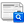## Journal of the European Optical Society - Rapid publications, Vol 5 (2010)

### Investigations on a robust profile model for the reconstruction of 2D periodic absorber lines in scatterometry

H. Gross, J. Richter, A. Rathsfeld, M. Bär

#### Abstract

Scatterometry as a non-imaging indirect optical method in wafer metrology is applicable to lithography masks designed for extreme ultraviolet (EUV) lithography , where light with wavelengths of about 13.5 nm is applied. The main goal is to reconstruct the critical dimensions (CD) of the mask, i.e., profile parameters such as line width, line height, and side-wall angle, from the measured diffracted light pattern and to estimate the associated uncertainties. The numerical simulation of the diffraction process for periodic 2D structures can be realized by the finite element solution of the two-dimensional Helmholtz equation. The inverse problem is expressed as a non-linear operator equation where the operator maps the sought mask parameters to the efficiencies of the diffracted plane wave modes. To solve this operator equation, the deviation of the measured efficiencies from the ones obtained computationally is minimized by a Gauss-Newton type iterative method. In the present paper, the admissibility of rectangular profile models for the evaluations of CD uniformity is studied. More precisely, several sets of typical measurement data are simulated for trapezoidal shaped EUV masks with different mask signatures characterized by various line widths, heights and side-wall angles slightly smaller than 90 degree. Using these sets, but assuming rectangular structures as the basic profiles of the numerical reconstruction algorithm, approximate line height and width parameters are determined as the critical dimensions of the mask. Finally, the model error due to the simplified shapes is analyzed by checking the deviations of the reconstructed parameters from their nominal values.

Full Text: PDF

#### Citation DetailsCite this article

#### References

M. Wurm, B. Bodermann, and W. Mirandé, "Evaluation of scatterometry tools for critical dimension metrology" DGaO Proc. 106, (2005).

H. Gross, R. Model,M. Bär, M. Wurm, B. Bodermann, and A. Rathsfeld, "Mathematical modelling of indirect measurements in scatterometry" Measurement 39, 782-794 (2006).

J. Perlich, F.-M. Kamm, J. Rau, F. Scholze, and G. Ulm, "Characterization of extreme ultraviolet masks by extreme ultraviolet scatterometry" J. Vac. Sci. Technol. B22, 3059-3062 (2004).

J. Pomplun, S. Burger, F. Schmidt, F. Scholze, C. Laubis, and U. Dersch, "Finite element analysis of EUV scatterometry" Proc. SPIE 6617, 18-21 (2007).

D. Colton, and R. Kress, Inverse Acoustic and Electromagnetic Scattering Theory (Second Edition, Springer-Verlag, New York 1998).

J. Elschner, and M. Yamamoto, "An inverse problem in periodic diffractive optics: Reconstruction of Lipschitz grating profiles" Appl. Anal. 81, 1307-1328 (2002).

H. Gross, A. Rathsfeld, F. Scholze, and M. Bär, "Profile reconstruction in extreme ultraviolet (EUV) scatterometry: modeling and uncertainty estimates" Meas. Sci.Technol. 20, 105102 (2009).

H. Gross, and A. Rathsfeld, "Sensitivity analysis for indirect measurement in scatterometry and the reconstruction of periodic grating structures" Wave. Random Complex 18, 129-149 (2008).

J. Elschner, R. Hinder, and G. Schmidt, "Finite element solution of conical diffraction problems" Adv. Comput. Math. 16, 139-156 (2002).

G. Bao, "Finite element approximation of time harmonic waves in periodic structures" SIAM J. Numer. Anal. 32, 1155-69 (1995).

J. Nocedal, and S. J. Wright, Numerical optimization (Springer Verlag, New-York, 2000).

R. M. Alassaad, and D. M. Byrne, "Error Analysis in Inverse Scatterometry I: Modeling" J. Opt. Soc. Am. A 24, 326-338 (2007).

DIPOG Homepage http://www.wias-berlin.de/software/DIPOG

O. Cessenat, and B. Depres, "Application of an ultra weak variational formulation of elliptic PDEs to the two-dimensional Helmholtz problem" SIAM J. Numer. Anal. 35, 255-299 (1998).

J. Pomplun, and F. Schmidt, "Reduced basis method for fast and robust simulation of electromagnetic scattering problems" Proc. SPIE 7390, 73900I (2009).

Joint Committee for Guides in Metrology BIPM, IEC, IFCC, ILAC, ISO, IUPAC, IUPAP, and OIML, "Evaluation of measurement data - Supplement 1" in Guide to the expression of uncertainty in measurement - Propagation of distributions using a Monte Carlo method (Bureau International des Poids et Mesures JCGM 101, 2008).

T. A. Germer, H. J. Patrick, R. M. Silver, and B. Bunday, "Developing an uncertainty analysis for optical scatterometry" Proc. SPIE 7272, 72720T (2009).

A. Kato, and F. Scholze, "The effect of line roughness on the reconstruction of line profiles for EUV masks from EUV scatterometry" Proc. SPIE 7636, 76362I (2010).